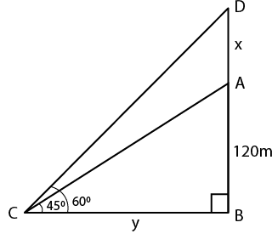Guru

# The angle of elevation of the top of an unfinished tower at a point distant 120 m from its base is 45. How much higher must the tower be raised so that its angle of elevation at the same point may be 60?

• 0

sir this is the important  question from the book -ML aggarwal( avichal publication) class 10th , chapter20 , heights and distances
The angle of elevation of the top of an unfinished tower
at a point distant 120 m from its base is 45.
How much higher must the tower be raised
so that its angle of elevation at the same point may be 60?

question no 36 , heights and distances , ICSE board, ML Aggarwal

Share

1. Consider AB as the unfinished tower where AB = 120 m

Angle of elevation = 450

Take x be higher raised so that the angle of elevation becomes 600

BC = yIn right triangle ABC

tan θ = AB/CB

Substituting the values

tan 450 = AB/CB = 120/y

So we get

1 = 120/y

y = 120 m

In right triangle DBC

tan 600 = DB/CB

Substituting the values

√3 = (120 + x)/ 120

120√3 = 120 + x

x = 120√3 – 120

x = 120 (√3 – 1)

So we get

x = 120 (1.732 – 1)

x = 120 (0.732)

x = 87.84 m

Hence, the tower should be raised at 87.84 m.

• 0